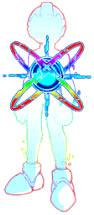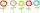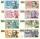# Unknown x

If we add to unknown number 21, then divide by 6 and then subtract 51, we get back an unknown number.

What is this unknown number?

x =  -57

### Step-by-step explanation:Did you find an error or inaccuracy? Feel free to write us. Thank you!Tips to related online calculators
Do you have a linear equation or system of equations and looking for its solution? Or do you have a quadratic equation?

## Related math problems and questions:

• Unknown numberSamuel wrote unknown number. Then he had add 200000 to the number and the result multiply by three. When it calculated he was surprised, because the result would have received anyway, if write digit to the end of original number. Find unknown number.
• Unknown number 24f we add 20, we get 50% of its triple. What is this unknown number?
• I think numberI think number.When I add 841 to it and subtract 157, I get a number that is 22 greater than 996. What number I thinking?
• Find unknown numberWhat is the number between 50 and 55 that is divisible by 2,3,6,9?
• MistakeNicol mistake when calculate in school. Instead of add number 20 subtract it. What is the difference between the result and the right result?
• Unknown number 5I think of an unknown number. If we enlarge it five times then subtract 3 and the result decreases by 75% we get one greater than the number. What number am I thinking of?
• One-thirdA one-third of unknown number is equal to five times as great as the difference of the same unknown number and number 28. Determine the unknown number.
• Unknown numberFind the unknown number equal to a quarter of a fifth of a number, which is by 152 more than an unknown number.
• DozenWhat is the product of 26 and 5? Write the answer in an Arabic numeral. Add up the digits. How many of this is in a dozen? Divide #114 by this
• RegroupingSubtract mixed number with regrouping: 11 17/20- 6 19/20
• Three 105Three consecutive integers add up to 51. what are these integers?
• Evaluate 11Multiply the quotient of 6 and 2 by 3, then add 1. Add 1 and 6, then divide by 2 and multiply by 3. Divide 6 by the product of 2 and 3, then add 1.
• NumbersDetermine the number of all positive integers less than 4183444 if each is divisible by 29, 7, 17. What is its sum?
• FlowersThe flower has six flowers, and each flower has a number. These are the numbers: 20,40,39,28,8,9. What number will be in the middle of the flower so that the numbers come from the flowers when we subtract and add?
• Cash backAfter a trip 24 students have left more than 650 but less than 690 CZK (Kc). How much each student receives return when the amount is divisible by the number of pupils?
• NumberCalculate the integer number which, divided by 34 gives 10 and the rest 25.
• Unknown number 716% of the unknown number is by 21 less than unknown number itself. Determine the natural unknown number.